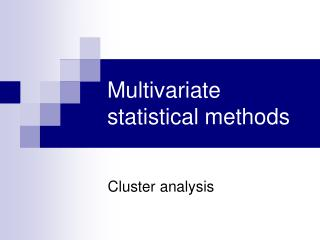Download PresentationMultivariate statistical methods

Multivariate statistical methods - PowerPoint PPT Presentation

Multivariate statistical methods. Cluster analysis. Multivariate methods. multivariate dataset – group of n objects, m variables (as a rule n > m, if possible). confirmation vs. eploration analysis confirmation – impact on parameter estimate and hypothesis testingI am the owner, or an agent authorized to act on behalf of the owner, of the copyrighted work described.
Download PresentationMultivariate statistical methods

Download Policy: Content on the Website is provided to you AS IS for your information and personal use and may not be sold / licensed / shared on other websites without getting consent from its author.While downloading, if for some reason you are not able to download a presentation, the publisher may have deleted the file from their server.

- - - - - - - - - - - - - - - - - - - - - - - - - - E N D - - - - - - - - - - - - - - - - - - - - - - - - - -
Presentation Transcript
1. Multivariate statistical methods Cluster analysis

2. Multivariate methods • multivariate dataset – group of n objects, m variables (as a rule n>m, if possible). • confirmation vs. eploration analysis • confirmation – impact on parameter estimate and hypothesis testing • exploration – impact on data exploration, finding out of patterns and structure

3. Multivariate statistical methods Unit classification • Cluster analysis • Discrimination analysis Analysis of relations among variables • Cannonical correlation analysis • Factor analysis • Principal component analysis

4. Unit classification methods

5. Cluster analysis (CA) • aim is find out groups of objects, which are similar and are different from other groups • methods of cluster analysis: • hierarchical • nonhierarchical

6. 1. Hierarchical methods • creation of clusters of different level (clusters of the highest level include clusters of lower level) • results of hierarchical methods are formed in tree structure, results are presented by dendrogram • is specified: • similarity rate • algorithms of clustering

7. Hierarchical methods – similarity expression • qualitative values • number of indentical values/number of all values • quantitative values: • Euclidean distance vzdálenost • Manhattan distance (Hemming distance) • Tschebyshev distance

8. Similarity rates • Euclidean distance • Manhattan (Hemming distance) • Tschebyshev distance where xik, xjk are objects, which distance is explored in n-dimension, n is number of observed characteristics

9. Distance of objects in 2D Distances: • Circle – Euclidean • Internal square – Hemming • External square – Tshebyshev

10. Other types of similarity rates • Power definied by user, the higher p is, the higher weight of larger distances is and it means lower signification of smaller distances. Parameter r causes conversely. • 1-Pearson r unsuitable for smal number of dimension • Percentual discrepancy suitable for categorical variables

11. Algoritms of clustering • Nearest neighbor linkage: distance between two clusters is definied as distance of two nearest objects • Furthest neighbor linkage: distance between two clusters is definied as distance of two furthest objects • Nonweighted group average linkage: distance between two clusters is definied as average distance among all of pairs, where 1st member is from 1st cluster and 2nd member is from 2nd cluster • Weighted group average linkage: as previous, extra takes note of cluster size (number of objects) as weights

12. Algorithms of clustering • Nonweighted centroid: distance between two clusters is definied as distance of centroids of these clusters. Centroid is vector of averages (each coordinate is average of appropriate coordinates of objects in the each cluster) • Weighted centroid: as previous,extra takes note of cluster size (number of objects) as weights • Ward´s method: different from previous, for computation of distance among clusters is used analysis of variance. For clustering is important this rule, that the internal cluster sum of squares is minimal

13. 2. Nonhierarchical method • mostly used is method K – means • algorithm is based on moving of objects among clusters • number of clusters is beforehand defined; randomly or according to experiences of analyst • centroids are defined for all clusters in the same step • all objects are explored. If the object is nearest to the own centroid, we leave it in this cluster. If not, move it in cluster, which centroid is the nearest. Intercluster sum of square should be minimal. This procedure repeat until at no objects shall be moved. Than we have final solution. • we are not working with distance matrix → K – means method is suitable for clustering of larger size of objects